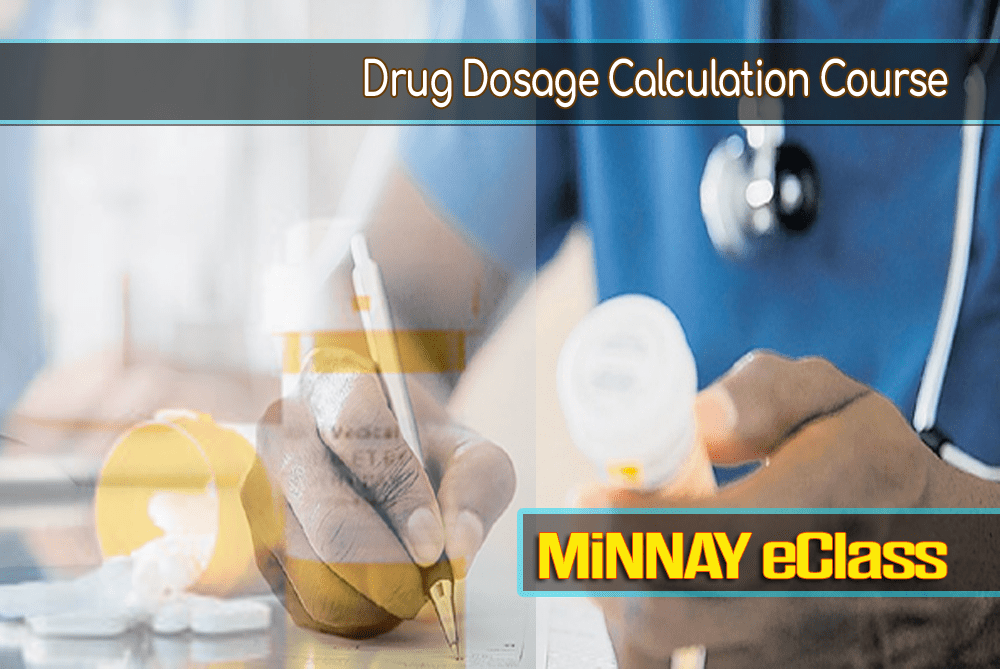﻿

# Drug Dosage and CalculationsDrug Dosage and Calculations Online Course with Exam ReviewsDrug dosage and calculation online course reviews the fundamental mathematical concepts used in drug dosage calculations. This course prepares the entry level nurse with a realistic approach for preparing dosages and solutions, including calculating intravenous flow rates and pediatric dosages. Topics include Roman numerals, fractions, decimals, percents, ratio and proportions, word problems, metrics, household and apothecary systems of measurement, equivalents, abbreviations, conversions, oral meds, parenteral meds, intravenous rates, and pediatric dosage calculations. This is a calculations class, not a foundational math course. Upon successful course completion, students will be able to apply basic mathematical concepts to calculate accurate dosage calculations.

Prerequisites: none

Learning Objectives:

1. Demonstrate competency in basic arithmetic function.
2. Solves problems using a critical thinking approach
3. Demonstrate the ability to convert between the metric, apothecary, and avoirdupois
systems.
4. Use Dimensional Analysis to calculate accurate dosages.
5. Demonstrate the ability to calculate dosages based on body weight of pediatric and

• Course Fee: \$79.99
• Course Category: Nursing Sciences
• Students Taken : 4078
• Lessons Included: 35
• Average Time to Completion: 21 hrs
• Overall Student Pass Rate: 99%

####LESSONS FOR DRUG DOSAGE CALCULATION COURSE

Section 1: Refresher Math
Chapter 1: Relative value, addition, and subtraction of decimals
Chapter 2: Multiplication and division of decimals
Chapter 3: Solving common fraction equations

Section 2: Introduction to Drug Measures
Chapter 4: Metric/International (SI) system
Chapter 5: Unit, percentage, milliequivalent, ratio, household, and apothecary, measures.

Section 3: Reading medication labels and syringe calibrations
Chapter 6: Oral medication labels and dosage calculations
Chapter 8: Hypodermic syringe measurements
Chapter 9: Parenteral medication labels and dosage calculation
Chapter 10: Reconstitution of powdered drugs
Chapter 11: Measuring insulin dosages

Section 4: Dosage Calculation
Chapter 12: Dosage calculation using dimensional

Section 5: Dosage calculations from body weight and surface area
Chapter 13: Adult and pediatric dosages based on body weight
Chapter 14: Adult and pediatric dosages based on body surface area [BSA]

Section 6: Intravenous calculations
Chapter 15: Introduction to IV therapy
Chapter 16: IV flow rate calculation
Chapter 17: Calculating IV infusion and completion times
Chapter 18: IV medication and titration calculations
Chapter 19: Heparin infusion calculations

Section 7: Pediatric Medication Calculations
Chapter 20: Pediatric oral and parenteral medications
Chapter 21: Pediatric intravenous medications

g

######1. Grade Score of 70% or more makes the participants eligible to Certificate of Achievement that can be downloadable as a PDF file.
2. A letter grade is also assigned on the Certificate of Achievement

##• None
• Certificate Level
• College/University Level

##• Self Study (No instructor)
• Instructor Available
• Instructor Request (If needed)

##• Online Courses
• Unlimited Accessibility for 6 and 12 months
• Start Anytime
• Self-Paced
• User Friendly
• Suitable to All Students
• PC & Mac Compatible
• Android/iOs Mobile and Tablet
• Class SurveysIf you already have an account with us,